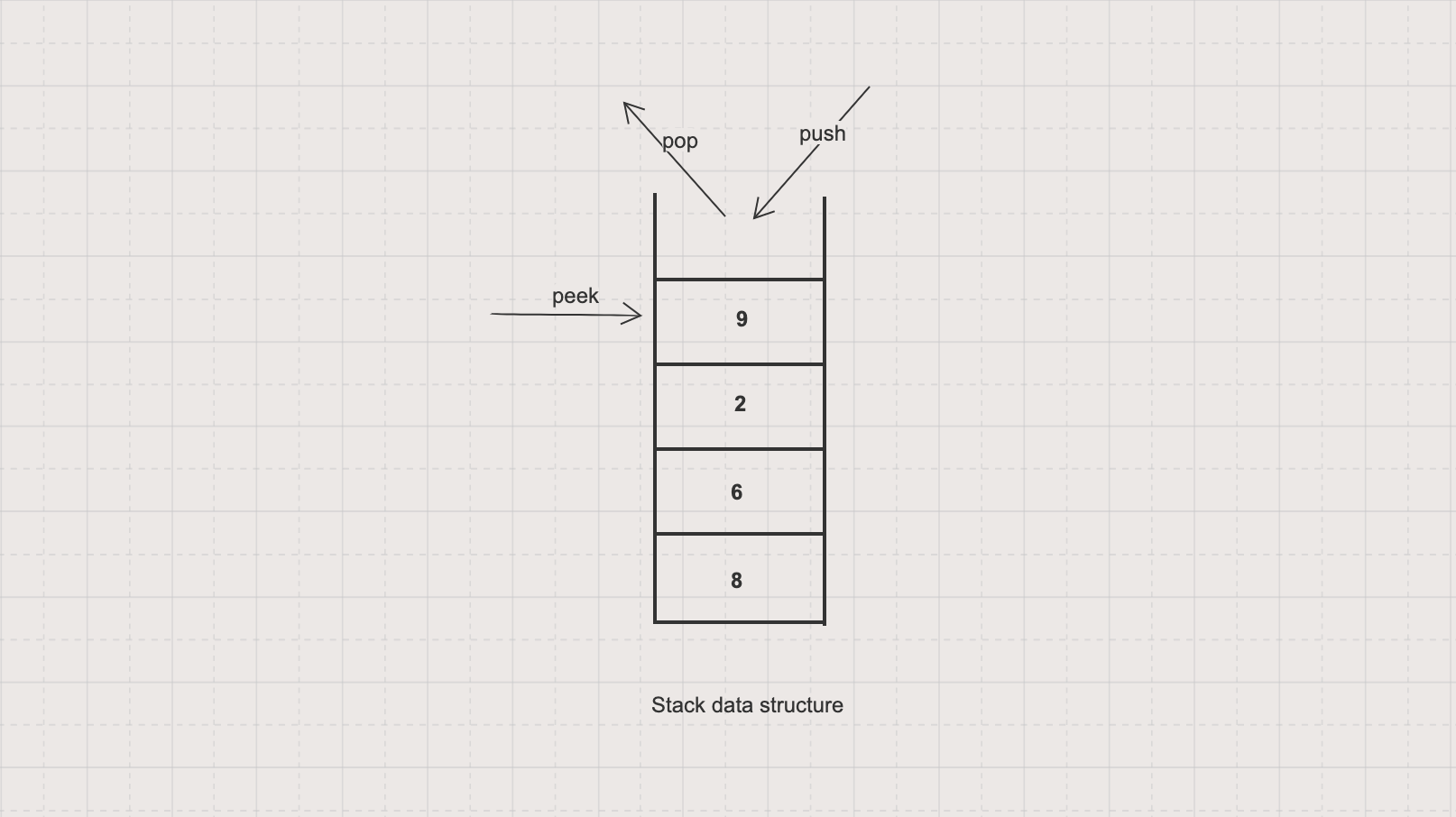Stack is a linear data structure consisting of items sorted in Last In First Out (LIFO) order due to adding or removing stack items is only possible at the topYou can think of a stack data structure similar to a stack of plates in real life

In this tutorial, we will learn to implement a `Stack` data structure and its operations with Java, including

• Push: adds an item onto a stack

• Pop: retrieves and removes the top item from a stack

• Peek: retrieves without removes the top item from a stack

We will also quickly walk through some official supports of Stack in Java such as `java.util.Deque`, `java.util.concurrent.BlockingDeque` and `java.util.Stack`

## Implementation

You can implement a stack by using either a Linked List or an Array Data Structure

The following is an implementation example using a static array

``````import java.util.NoSuchElementException;

public class StackByArray {
private int[] stack;
private int top;

public StackByArray(int capacity) {
stack = new int[capacity];
top = -1;
}

public void push(int x) {
if (isFull()) {
throw new IllegalStateException();
}

stack[++top] = x;
}

public int pop() {
if (isEmpty()) {
throw new NoSuchElementException();
}

return stack[top--];
}

public int peek() {
if (isEmpty()) {
throw new NoSuchElementException();
}

return stack[top];
}

public boolean isEmpty() {
}

public boolean isFull() {
}

public int size() {
}

public static void main(String[] args) {
StackByArray myStack = new StackByArray(3);
myStack.push(4);
myStack.push(2);
myStack.push(5);

System.out.println(myStack.peek()); // 5
System.out.println(myStack.pop()); // 5
System.out.println(myStack.peek()); // 2

System.out.println(myStack.size()); // 2
System.out.println(myStack.isEmpty()); // false
System.out.println(myStack.isFull()); // false
}
}
``````

## Application

• Depth First Search uses a stack to track which elements to visit next

## Stack implementations in Java

• `java.util.Deque` (interface), since Java 1.6, unsynchronized / not thread-safe, using in single threaded environment. For example
``````Deque<Integer> stack = new ArrayDeque<>();
stack.push(1);
stack.peek();
stack.pop();
``````
• `java.util.concurrent.BlockingDeque` (interface), since Java 1.6, synchronized / thread-safe, using in multi threaded environment. For example
``````BlockingDeque<Integer> stack = new LinkedBlockingDeque<>();
stack.push(1);
stack.peek();
stack.pop();
``````
• `java.util.Stack` (class), since Java 1.1, extends `Vector`, synchronized, should not be used due to its negative impact on performance